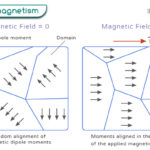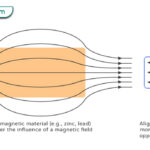Home / Physics / Kinetic Friction

# Kinetic Friction

## What is Kinetic Friction

An object that moves over a surface experiences resistance to its motion. This resistance is known as kinetic friction. Also called dynamic friction, the frictional force is in a direction opposite to the object’s motion. Kinetic friction occurs when two surfaces are in relative motion to each other. It takes place at the point of contact between the two surfaces, and the kinetic energy gets converted into heat.

## Types of Kinetic Friction

There are two types of kinetic friction based on the motion of the object. Examples of both these types can be observed in daily life.

### Sliding Friction

In this type of friction, the object slides over the surface.

Examples

• A vehicle skidding on the road after applying brakes
• An ice skater or a skier coming to a stop
• A box being pushed on a floor
• Generation of heat due to rubbing of hands

### Rolling Friction

In this type of friction, the object rolls over the surface

• A soccer ball coming to a stop after kicking it
• A golf ball coming to a stop after rolling over the green
• A roller skater coming to a stop
• A roller coaster slowing down before coming to a stop

## Laws of Kinetic Friction

Here are some laws that the kinetic friction follows.

• Acts in a direction opposite to the object’s motion
• Proportional to the normal force
• Independent of the area of contact between the surfaces
• Independent of the sliding speed as long as the speed is not too high

## Kinetic Friction Equation

According to the laws of kinetic friction, the frictional force FK is proportional to the normal force FN. Therefore, the magnitude of kinetic friction is given by

FK FL

Or, FK = μL FL

Symbol of kinetic friction: FK

Unit of kinetic friction: Newton or N

Dimension of kinetic friction: MLT-2

The constant of proportionality μL is called the coefficient of kinetic friction. It is unitless and dimensionless. Its value depends upon the pair of surfaces. The coefficient of kinetic friction is less than that of static friction.

The work done by a force is the product of the force and displacement d. Therefore, the work done W by the kinetic friction force is

W = FK x d

The kinetic friction remains constant over time as long as the normal load remains unchanged.

### How to Find the Coefficient of Kinetic Friction

The coefficient of kinetic friction can be determined through experiments, such as measuring the force needed to overcome friction or measuring the angle at which an object will start to slide off an incline.

Article was last reviewed on Wednesday, December 2, 2020

### Related articlesWave-Particle DualityPhotoelectric EffectFerromagnetismDiamagnetism